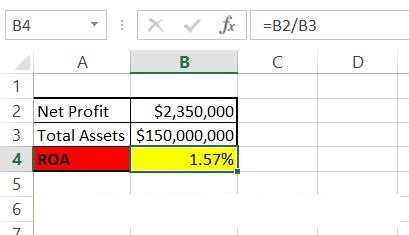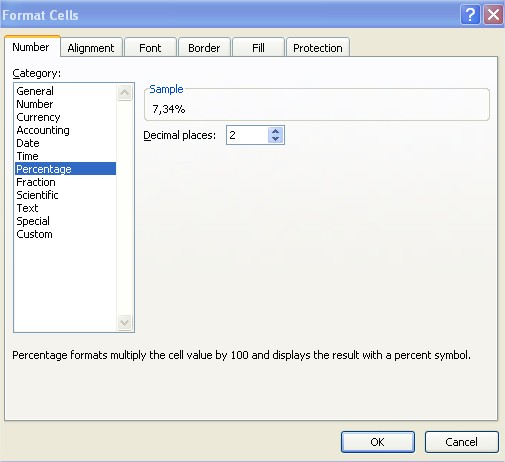### How to calculate ROA in Excel

In this lesson you can learn how to calculate ROA in Excel.

ROA stands for return on assets. Return of assets is one of the most basics measures in the business. ROA is an indicator which is used to measure the efficiency of the company.

Let’s build the return on assets calculator in Excel. To calculate ROA in Excel first you need some data. You need net profit and total assets.

Copy and paste this roi formula in cell B4: =B2/B3This formula will calculate the ROA for the assets data you place in cells B2 and B3 and is based on roa equation formula:

ROA = Net Profit / Total Assets

Remember to format ROA as Percentage. Click B4 cell > click CTRL + 1 keyboard shortcut > click Percentage with 2 decimal places## Template

```Further reading: